TT Order Types

# TT Autohedger

TT Autohedger allows traders to programmatically hedge their options trades with the underlying instrument. The TT Autohedger order type is used when submitting an order for an options instrument. When the options order fills, TT Autohedger uses the total delta of the executed options trade to calculate an order quantity for the underlying instrument. This helps traders maintain a delta neutral position.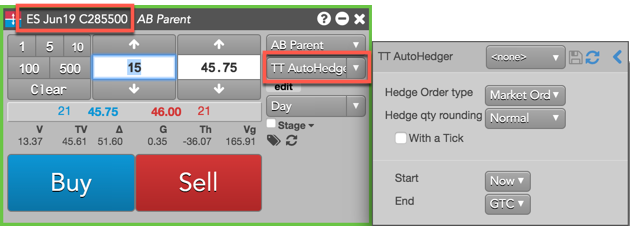## TT Autohedger behavior

After a fill is received for the options order, TT Autohedger submits a Market order for the underlying futures instrument with a quantity equal to: (fill quantity * delta). If delta is a positive value, a Sell order is submitted. If delta is negative, a Buy order is submitted. If delta is "0", no hedge order is submitted. TT Autohedger continuously receives updates to delta based on the current market.

By default, TT Autohedger uses the calculated hedge order quantity with normal rounding. However, you can also configure TT Autohedger to either round up or round down the value. For example, a hedge quantity between 96.01 — 97.00 is rounded up to "97" or rounded down to "96.00".

### Calculating the hedge order quantity: Normal setting

In this example, Hedge qty rounding is set to the default setting of "Normal" and delta for the options instrument is "96.77". The initial options order is submitted for 15 contracts.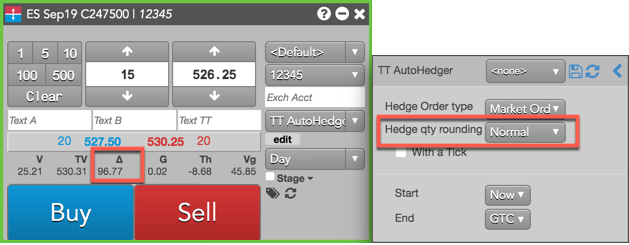When the 15 lot order is filled, TT Autohedger multiplies delta for the instrument by the fill quantity of "15" for the ES Sep19 C247500 Call contract to determine the hedge order quantity. Delta is displayed as whole number, but is actually a percentage.

Hedge order quantity = 15 x.9677 = 14.5155 = 15 futures.

### Calculating the hedge order quantity: Round Up setting

In this next example, Hedge qty rounding is set to "Round Up" when buying 15 of the ES Sep19 C247500 contracts. When TT Autohedger multiplies the options fill quantity by delta, the result will be rounded up.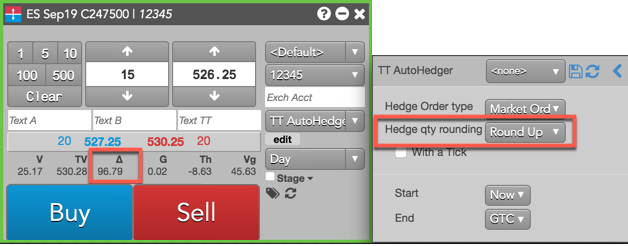Hedge order quantity = 15 X .9679 = 14.5185 = 15 futures.

### Calculating the hedge order quantity: Round Down setting

In this last example, Hedge qty rounding is set to "Round Down" when buying 15 of the ES Sep19 C247500 contracts. When TT Autohedger multiplies the options fill quantity by delta, the result will be rounded down.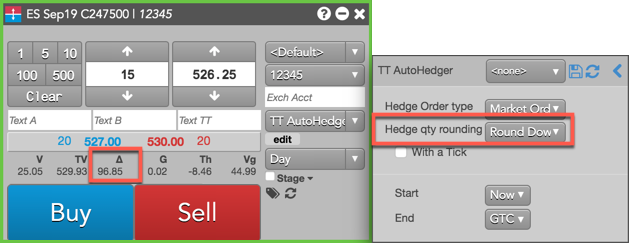Hedge order quantity = 15 X .9685 = 14.5275 = 14 futures.

## TT Autohedger configuration

When configuring an order for an options instrument in MD Trader or the Order Ticket and the TT Autohedger order type is selected, the configuration fly-out opens.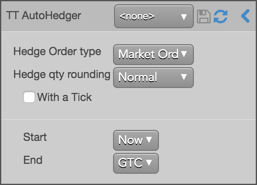The following parameters are included:

• Hedge Order type — Market order only
• Hedge qty rounding — Allows you to round delta for the underlying hedge order. Select one of the following settings:

• Normal: Uses delta with rounding when calculating the underlying order quantity (default setting)
• Round Down: Rounds down delta when calculating the underlying order quantity
• Round Up: Rounds up delta when calculating the underlying order quantity
• With a Tick: Sets the threshold to reprice the order.

The quantity can be specified as:
• Qty for an absolute number of contracts
• % for a percentage of the initial quantity for this order
• Start: Sets the date and time to start executing the order.

Values include:

• Now to start the order immediately
• Time to display a date/time picker for you to indicate when to start the order
• Pre-open to enter the order at the pre-open state defined by an exchange
• Open to enter the order when the exchange opens its trading session
• End: Sets the time to stop executing the logic of the order

Possible values include:
• GTC, which leaves the order working until canceled
• Time, which displays a date/time picker for you to indicate when to stop the order
• Day, which leaves the order working until the market closes

If End Time is selected and the End Time is reached, the order is deleted and the specified End Action is applied to its child orders. If the trading session is closed when the End Time is reached, the delete request will fail, leaving working GTC child orders on the exchange. It is your responsibility to delete these orders when the exchange re-opens.

## Entering a TT Autohedger order

To enter a TT Autohedger order:

1. Open MD Trader® or Order Ticket for an options outright contract.
2. Enter the order quantity and price for the order.
3. Select TT Autohedger as the order type.
4. Enter the TT Autohedger order parameters.
5. Click Buy or Sell to submit the order.